# 尺寸链公差分析｜线性尺寸链计算背后的数学逻辑

--毕达哥拉斯

**********************************************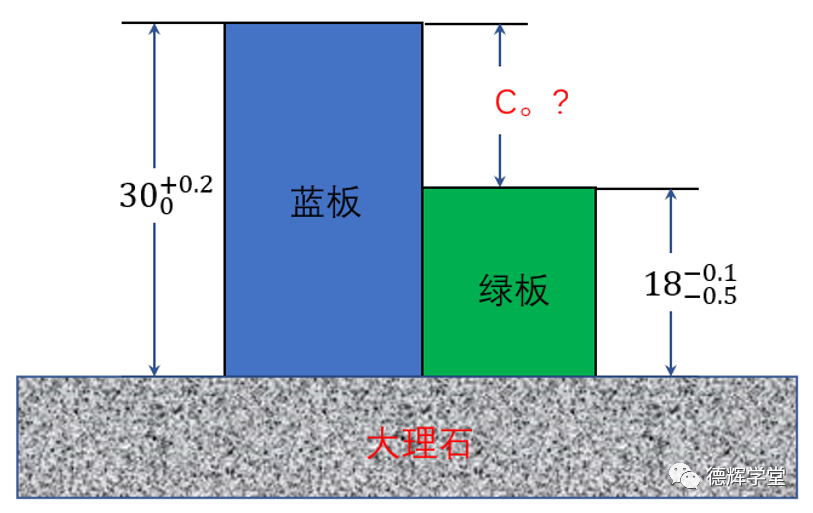D_C0=30.1-17.7=12.4

T_C0=±（0.1+0.2）=±0.3

C0=12.4±0.3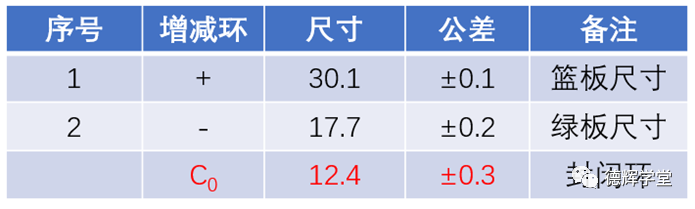1. 矢量累加和尺寸累加

2. 区间分析和公差累加

1.    矢量累加和尺寸累加

1）矢量和矢量累加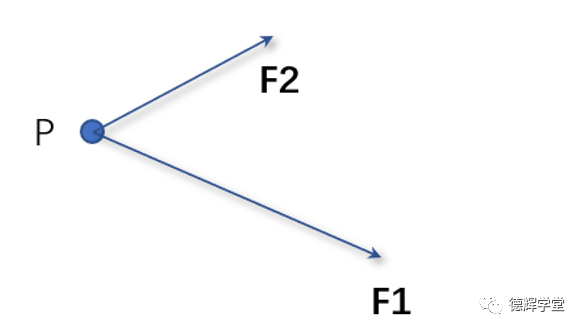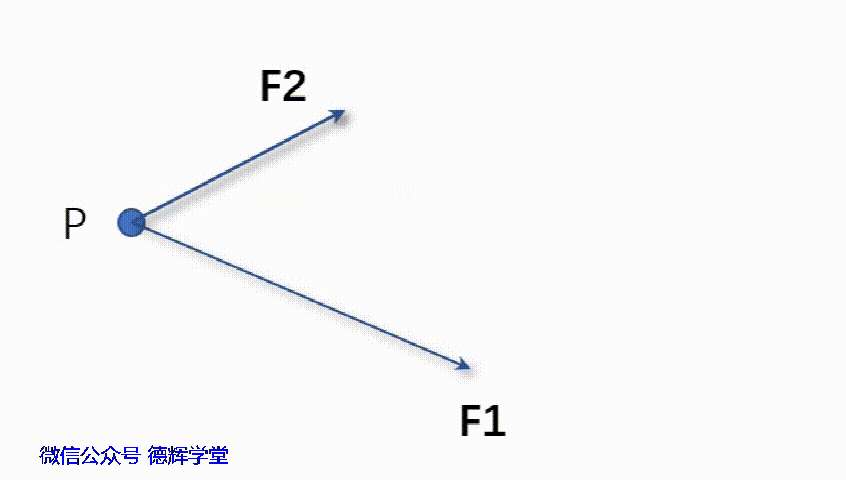F3 = F1 F2’    (1)

F3 = F1 + F2    (2)

1. 为啥矢量可以任意平移？

2. 如果力的大小不能直接相加的话，那公式（2）对计算合力来说有啥意义？

（注意，我们讨论的自由矢量，可以任意平移，但是有些矢量，比如位置矢量，线矢量，旋量等，和位置相关的，不能随便移动的）。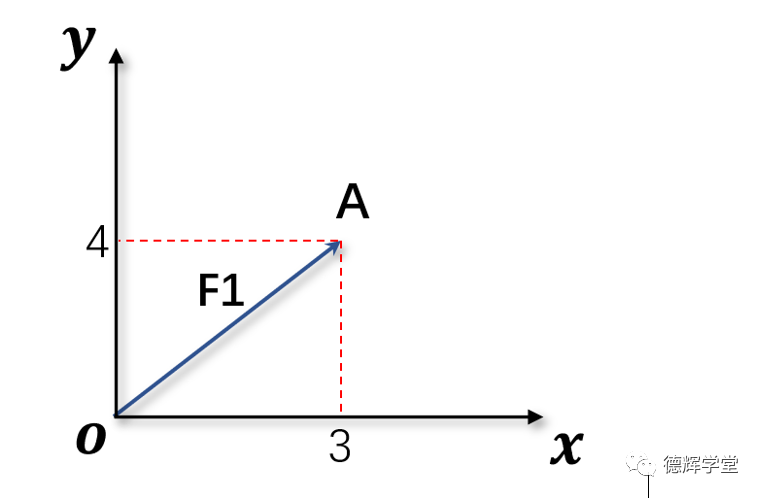F1 = OA (3,4)

F1 = OA = 3i+4j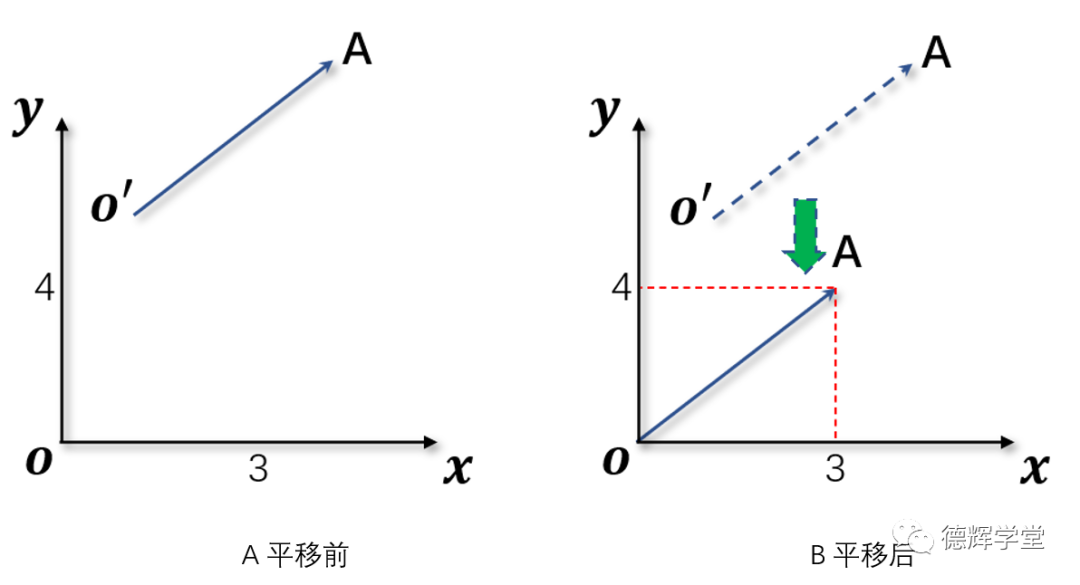O’A = OA = (3,4) = 3i+4j

|O’A| = |OA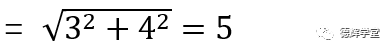矢量的模很重要，因为我们算尺寸链的时候，无论是缝隙，面差，过盈量之类的，我们关心的是大小(矢量的模)，而矢量本身我们不太关心。

F1+F2 = (a+c)i+(b+d)j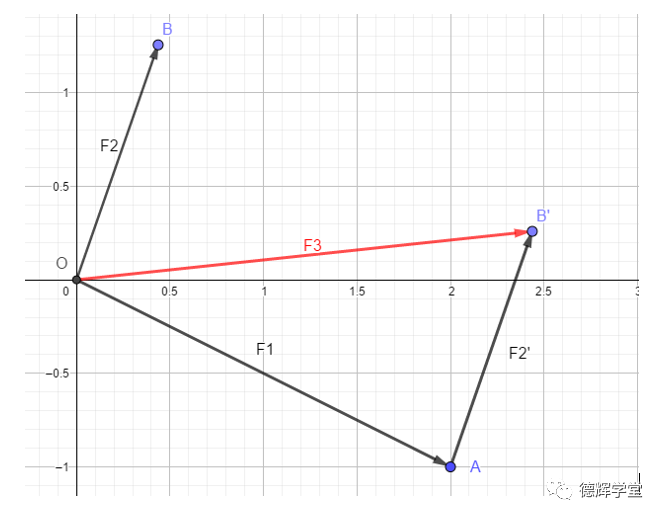F3 = F1 + F2 = OB’

F3 = F1 + F2 = （2i-j）+（0.44i+1.26j

=(2+0.44)i+(-1+1.26)j

=2.44i+0.26j

|F3|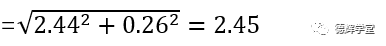搞定。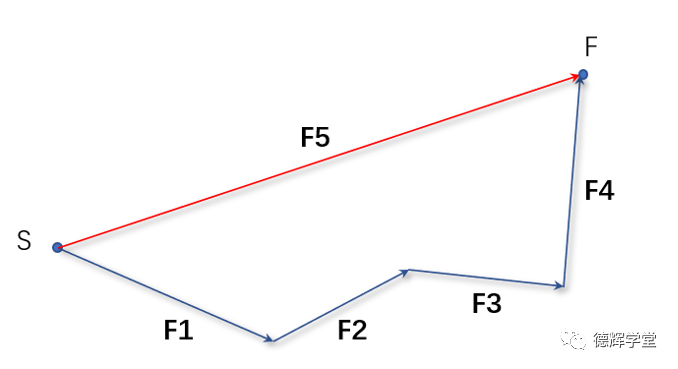F5 = F1 + F2 + F3 +F4

1. 矢量之和可以用三角形法作图表达，实际上就是多个矢量首尾相连叠加后的结果。比如图7中的红色矢量，它表达了矢量叠加的几何意义;

2. 矢量可以用坐标表达，或者用代数表达，本质上是一样的。比如矢量F1既可以表达成F1=（3 , 4），也可以表达成 F1 = 3+ 4，（当然，本期文章用的2D的案例，3D的话，再增加一个Z坐标即可）;

3. 矢量相加，实际上就是多个矢量对应的XYZ坐标分量分别相加。

现在我们进入第二小节。

2）矢量累加和尺寸累加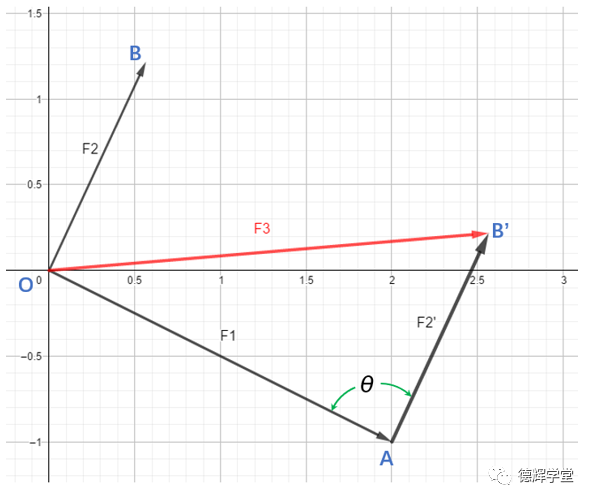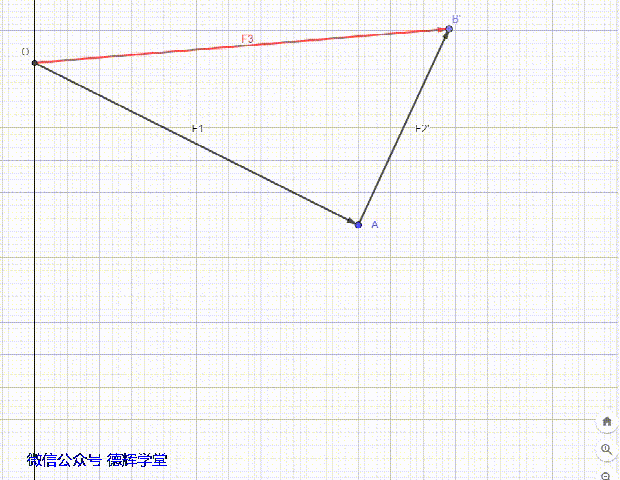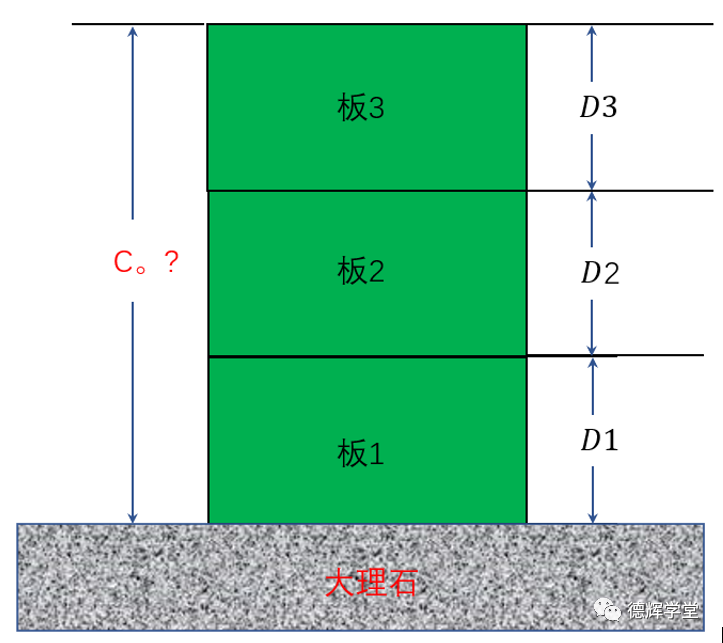C0 = D1 + D2 + D3   （3）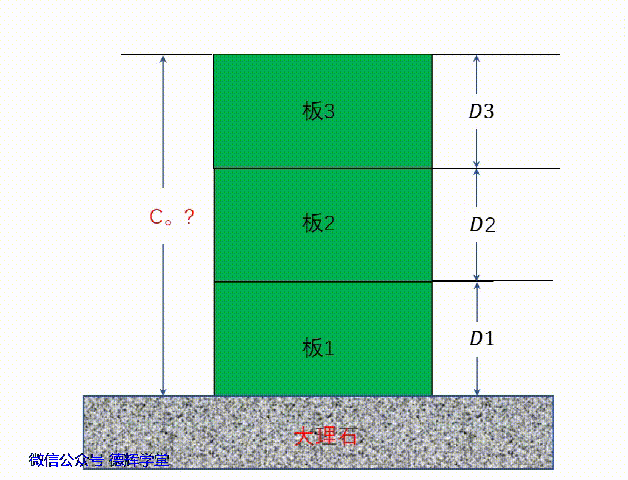F1 = (0,D1) = 0i+D1=D1 j

F2 = (0,D2) = 0i+D2=D2 j

F3 = (0,D3) = 0i+D3=D3 j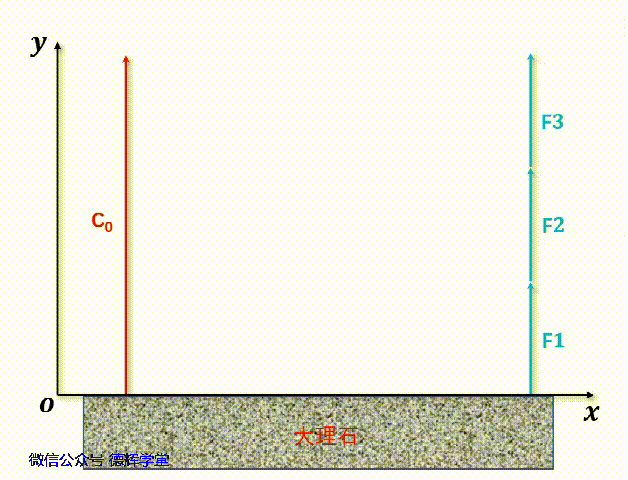C= F1 + F2 +F3

Cxi + Cyj = D1+ D2+ D3j

Cxi + Cyj = 0i + (D1+ D2 + D3) j

| C0| = D1+D2+D3     (4)

（这里就不在啰嗦勾股定理了，一条边的长度是0哦）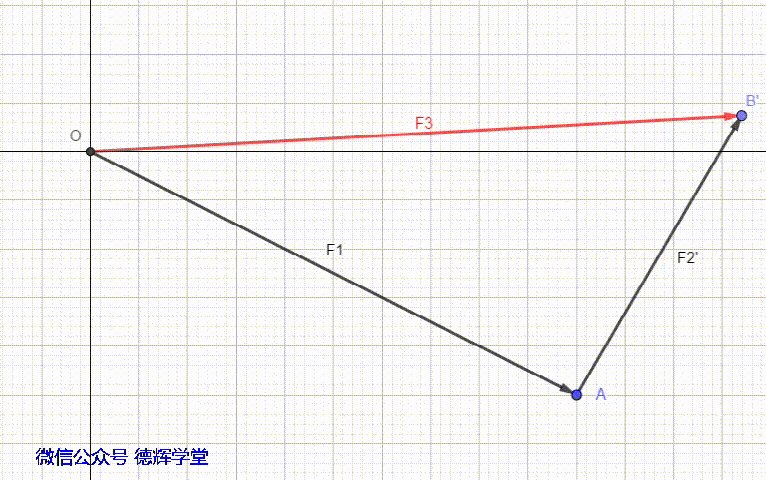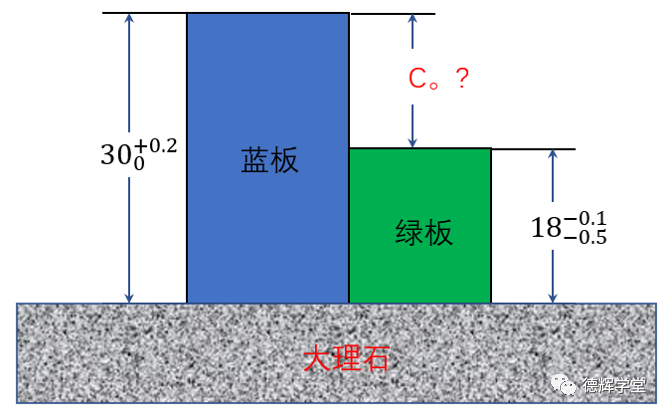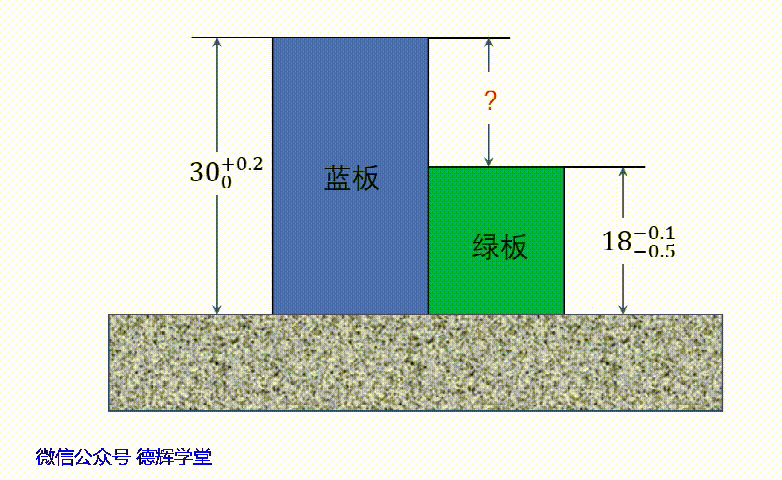D1 =（0, 30+0.2/0）= （30+0.2/0）j

D2 =（0, -18-0.1/-0.5

= -（18-0.1/-0.5）j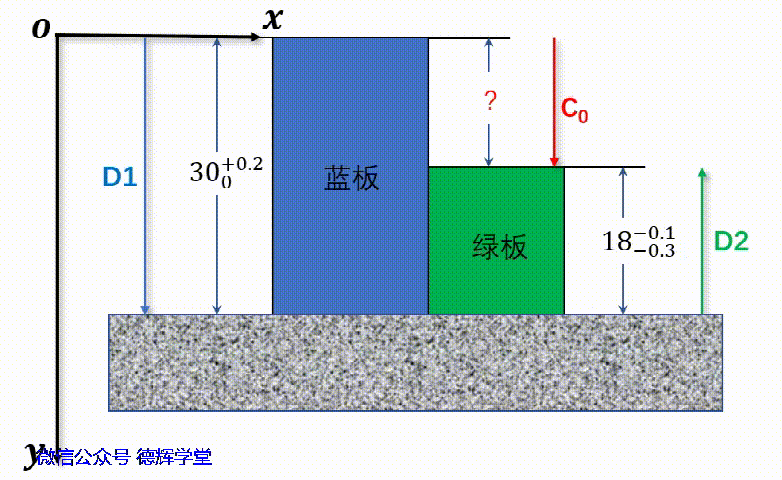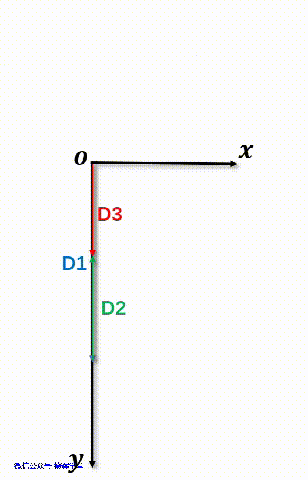CD1 + D2

=（30+0.2/0）+(-(18-0.1/-0.5）) j

=(（30+0.2/0）-（18-0.1/-0.5）) j

|C0=(30+0.2/0)-(18-0.1/-0.5) （5）

1. 任何尺寸链的计算，都可以用矢量和来表达，也就是可以用首尾相连的矢量图来表示，组成环就是这些矢量，而矢量之和就是封闭环。

2. 对于一般的线性尺寸链，大部分是单维度的（一维），要么只有X轴的分量，要么只有Y轴的分量，这些分量的大小就是具体的尺寸，所以计算起来非常简单。

3. 因为尺寸链必须表达成首尾相连的矢量和，在一维的尺寸链里边，有可能组成环之间的矢量方向会相反，这样就会产生减环。

4. 判断增减环的方法，和建坐标系有关系，一般的建议是，把封闭环的方向设置成坐标轴的正方向，然后看组成环的方向，和封闭环方向相同的是增环，相反的就是减环。当然，在计算的时候，增环用加法，减环用减法。

2.    区间分析和公差累加

1）区间数学的基本知识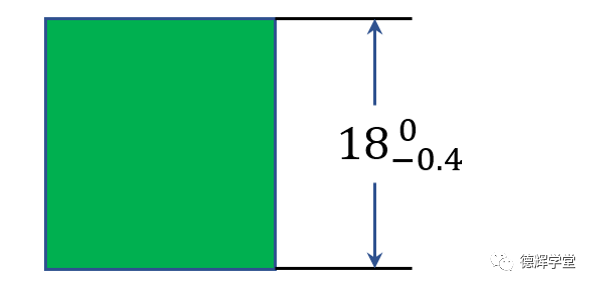X∈[17.6 , 18]

1. 区间的加法

X+Y = [ a+c , b+d ]

X + Y = [ 14 , 16 ]

2. 区间的减法

X-Y = [ a-d , b-c ]

X - Y = [ 3 , 5 ]

3. 区间的中点和宽度

m(x)=(a+b)/2,  w(x)=b-a

4. 点区间和对称区间

X = [ a , a ] = a

X + Y = [ -a-b , a+b ] =（a+b）[-1, 1]

X - Y = [ -a-b , a+b ] =（a+b）[-1, 1]

X =a[-1, 1]   (6)

Y =b[-1, 1]   (7)

X+Y-Z = (a+b+c) [-1, 1]

X-Y+Z = (a+b+c) [-1, 1]

X = [ a, b ] = m(x)+w(x)/2[ -1, 1 ]

= (a+b)/2 + (b-a)/2[ -1, 1]   （8）

X=(a+b)/2 + (b-a)/2[ -1, 1 ]

= [ (a+b)/2, (a+b)/2] + [ -(b-a)/2,(b-a)/2]

= [ (a+b)/2-(b-a)/2,(a+b)/2+(b-a)/2]

= [ a, b ]

2）区间分析和公差累加

D = [ 10, 10.2 ]

D =10.1 + 0.1[ -1, 1 ]     (9)

D = 10.1±0.1   （10）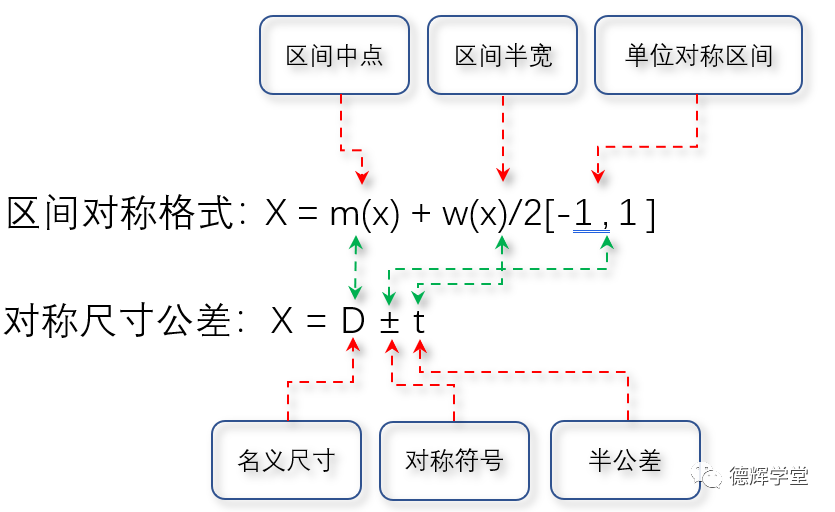C= X1 + X2 – X3

= (D1±t1) + (D2±t2) – (D3±t3)

C= (D1±t1) + (D2±t2) – (D3±t3)

=（D1+D2-D3）±（t1+t2+t3）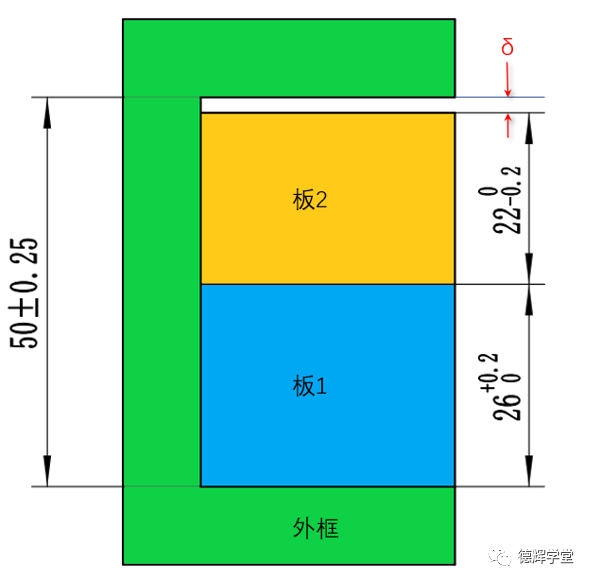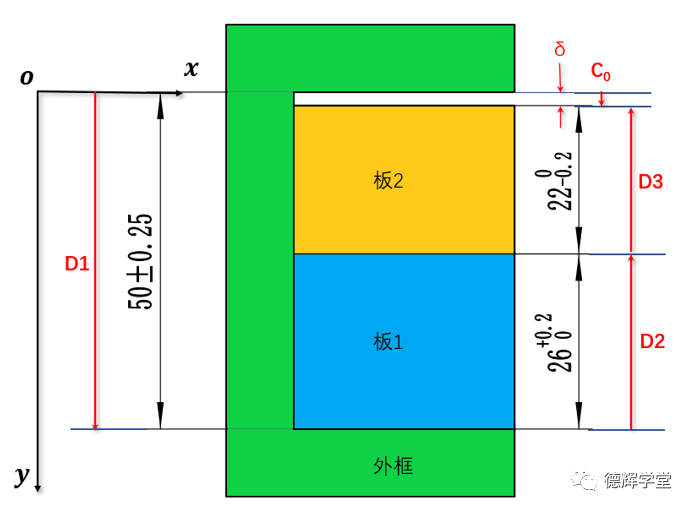C= D1 + D2 + D3

D1 = ( 0, 50±0.25 ) =（50±0.25）j

D2 = ( 0, -260+0.2) = -(260+0.2j

D3 = ( 0, -220-0.2) = -(220-0.2j

C= D1 + D2 + D3

=（50±0.25）- (260+0.2- (220-0.2)j

=（50±0.25 - 260+0.2  - 220-0.2j

δ = |C0| = 50±0.25 - 260+0.2  - 220-0.2

δ = 50±0.25 – 26.1±0.1– 21.9±0.1

=（50-26.1-21.9）±（0.25+0.1+0.1）

= 2 ± 0.45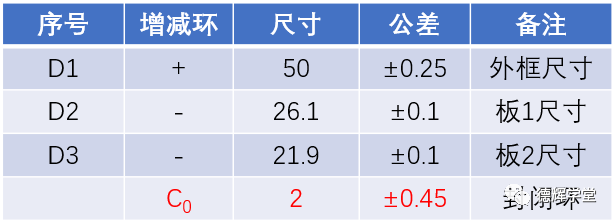本期文章的目的，本质上是在为我们平时计算尺寸链累加所用的方法寻求理论依据，证明其合法性。相当于强行扯过来一顶光鲜的帽子戴在头上，表示其光鲜。

1. 同济大学数学系 高等数学第七版上下册 高等教育出版社 2008

2. Moore, R., 1979, ‘‘Methods and Applications of Interval Analysis,’’ Society for Industrial and AppliedMathematic, ISBN 0898711614• ### CQI-9热处理系统评估 第四版

上海 12月-06-08日

• ### 高级先期产品质量策划（高级APQP）

上海 12月-11-13日

• ### CQI-17锡焊系统评估 第二版

上海 12月-18-20日

• ### 尺寸链计算和公差叠加（美国ASME认证高级专家授课）

上海 12月-28-29日

• ### CQI-15焊接系统评估 第二版

上海 12月-28-29日

### 更多热门资讯

23号C座B36,邮编：200023

### 客户反馈FeedbackCopyRight © 冰衡咨询 版权所有 沪ICP备18032273号-4本站由提供CDN加速服务